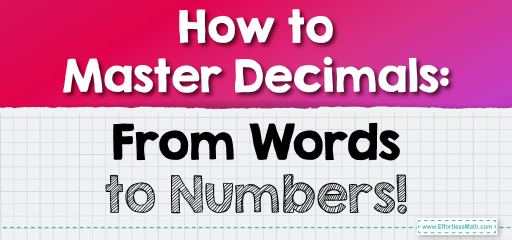# How to Master Decimals: From Words to Numbers!

Decimals are a way to represent parts of a whole. They are especially useful when we want to talk about values that are less than one whole unit. In our daily lives, we often use decimals when dealing with money, measurements, and more. But sometimes, decimals are conveyed in words rather than numbers. In this post, we'll learn how to convert these worded decimals into their numerical form.## Understanding Decimals Conveyed in Words

### Example 1:

Worded Decimal: “Three and four tenths.”

Numerical Form: To convert this into a number, we start with the whole number, which is $$3$$. Next, we look at the decimal part, “four-tenths.” This means $$4$$ out of $$10$$, which is written as $$0.4$$. So, the numerical form is $$3.4$$.

The Absolute Best Book for 5th Grade Students

1. Write down the whole number: $$3$$.

2. Convert “four tenths” to $$0.4$$.

3. Combine the whole number and the decimal: $$3.4$$.

### Example 2:

Worded Decimal: “Five and seventy-six hundredths.”

Numerical Form: Start with the whole number, $$5$$. The decimal part, “seventy-six hundredths,” means $$76$$ out of $$100$$. This is written as $$0.76$$. So, the numerical form is $$5.76$$.

1. Write down the whole number: $$5$$.

2. Convert “seventy-six hundredths” to $$0.76$$.

3. Combine the whole number and the decimal: $$5.76$$.

Remember, understanding how to convert worded decimals into their numerical form is a valuable skill, especially when dealing with real-world situations like shopping or measuring. Practice regularly to master this concept!

### Practice Questions:

1. Convert the worded decimal “Two and three-tenths” into its numerical form.

2. Convert “Seven and forty-five hundredths” into its numerical form.

3. Convert “Zero and nine tenths” into its numerical form.

4. Convert “Four and twelve hundredths” into its numerical form.

5. Convert “Six and eight tenths” into its numerical form.

A Perfect Book for Grade 5 Math Word Problems!

1. $$2.3$$

2. $$7.45$$

3. $$0.9$$

4. $$4.12$$

5. $$6.8$$

The Best Math Books for Elementary Students

### What people say about "How to Master Decimals: From Words to Numbers! - Effortless Math: We Help Students Learn to LOVE Mathematics"?

No one replied yet.

X
51% OFF

Limited time only!

Save Over 51%

SAVE $15 It was$29.99 now it is \$14.99